### 8.5.2.3 Obtaining Dijkstra-like algorithms

For motion planning problems, it is expected that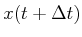, as computed from (8.62), is always close to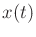relative to the size of. This suggests the use of a Dijkstra-like algorithm to compute optimal feedback plans more efficiently. As discussed for the finite case in Section 2.3.3, many values remain unchanged during the value iterations, as indicated in Example 2.5. Dijkstra's algorithm maintains a data structure that focuses the computation on the part of the state space where values are changing. The same can be done for the continuous case by carefully considering the sample points .

During the value iterations, there are three kinds of sample points, just as in the discrete case (recall from Section 2.3.3):

1. Dead: The cost-to-go has stabilized to its optimal value.
2. Alive: The current cost-to-go is finite, but it is not yet known whether the value is optimal.
3. Unvisited: The cost-to-go value remains at infinity because the sample has not been reached.
The sets are somewhat harder to maintain for the case of continuous state spaces because of the interaction between the sample set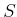and the interpolated region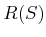.

Imagine the first value iteration. Initially, all points in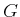are set to zero values. Among the collection of samples, how many can reach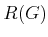in a single stage? We expect that samples very far fromwill not be able to reach; this keeps their values are infinity. The samples that are close toshould reach it. It would be convenient to prune away from consideration all samples that are too far fromto lower their value. In every iteration, we eliminate iterating over samples that are too far away from those already reached. It is also unnecessary to iterate over the dead samples because their values no longer change.

To keep track of reachable samples, it will be convenient to introduce the notion of a backprojection, which will be studied further in Section 10.1. For a single state,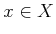, its backprojection is defined as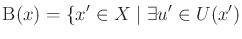such that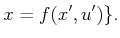(8.66)

The backprojection of a set,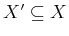, of points is just the union of backprojections for each point: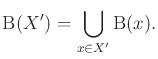(8.67)

Now consider a version of value iteration that uses backprojections to eliminate some states from consideration because it is known that their values cannot change. Letrefer to the number of stages considered by the current value iteration. During the first iteration,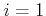, which means that all one-stage trajectories are considered. Letbe the set of samples (assuming already that none lie in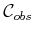). Let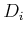and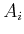refer to the dead and alive samples, respectively. Initially,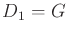, the set of samples in the goal set. The first set,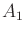, of alive samples is assigned by using the concept of a frontier. The frontier of a set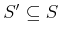of sample points is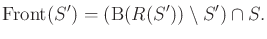(8.68)

This is the set of sample points that can reach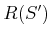in one stage, excluding those already in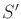. Figure 8.22 illustrates the frontier. Using (8.68),is defined as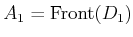.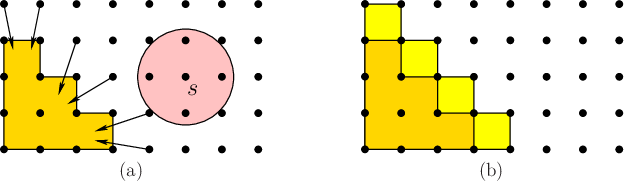Now the approach is described for iteration. The cost-to-go update (8.56) is computed at all points in. If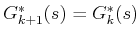for some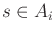, then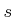is declared dead and moved to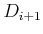. Samples are never removed from the dead set; therefore, all points inare also added to. The next active set,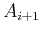, includes all samples in, excluding those that were moved to the dead set. Furthermore, all samples in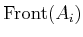are added tobecause these will produce a finite cost-to-go value in the next iteration. The iterations continue as usual until some stage,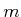, is reached for which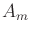is empty, and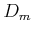includes all samples from which the goal can be reached (under the approximation assumptions made in this section).

For efficiency purposes, an approximation to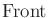may be used, provided that the true frontier is a proper subset of the approximate frontier. For example, the frontier might add all new samples within a specified radius of points in. In this case, the updated cost-to-go value for somemay remain infinite. If this occurs, it is of course not added to. Furthermore, it is deleted fromin the computation of the next frontier (the frontier should only be computed for samples that have finite cost-to-go values).

The approach considered so far can be expected to reduce the amount of computations in each value iteration by eliminating the evaluation of (8.56) on unnecessary samples. The same cost-to-go values are obtained in each iteration because only samples for which the value cannot change are eliminated in each iteration. The resulting algorithm still does not, however, resemble Dijkstra's algorithm because value iterations are performed over all ofin each stage.

To make a version that behaves like Dijkstra's algorithm, a queue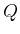will be introduced. The algorithm removes the smallest element ofin each iteration. The interpolation version first assigns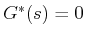for each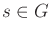. It also maintains a set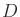of dead samples, which is initialized to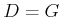. For each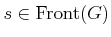, the cost-to-go update (8.56) is computed. The priorityis initialized to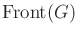, and elements are sorted by their current cost-to-go values (which may not be optimal). The algorithm iteratively removes the smallest element from(because its optimal cost-to-go is known to be the current value) and terminates whenis empty. Each time the smallest element,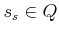, is removed, it is inserted into. Two procedures are then performed: 1) Some elements in the queue need to have their cost-to-go values recomputed using (8.56) because the value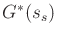is known to be optimal, and their values may depend on it. These are the samples inthat lie in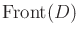(in whichjust got extended to include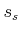). 2) Any samples in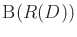that are not inare inserted intoafter computing their cost-to-go values using (8.56). This enables the active set of samples to grow as the set of dead samples grows. Dijkstra's algorithm with interpolation does not compute values that are identical to those produced by value iterations because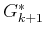is not explicitly stored when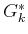is computed. Each computed value is some cost-to-go, but it is only known to be the optimal when the sample is placed into. It can be shown, however, that the method converges because computed values are no higher than what would have been computed in a value iteration. This is also the basis of dynamic programming using Gauss-Seidel iterations .

A specialized, wavefront-propagation version of the algorithm can be made for the special case of finding solutions that reach the goal in the smallest number of stages. The algorithm is similar to the one shown in Figure 8.4. It starts with an initial wavefront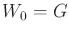in whichfor each. In each iteration, the optimal cost-to-go valueis increased by one, and the wavefront,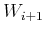, is computed from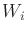as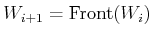. The algorithm terminates at the first iteration in which the wavefront is empty.

Steven M LaValle 2020-08-14